##### The Japanese Bonsai specialist
Direct order Contact Help / Services Newsletter# Spray attachment for soda bottle

› Bonsai tools › Watering equipment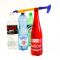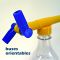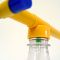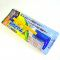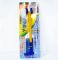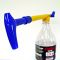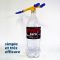ref. : 6874

12,80

Available quantity : 1Order

###### Description

Measurements: handles 70 mm, total length 350 mm. A convenient and economic atomiser that screws onto soda bottles and pumps a fine and delicate spray from its two spouts.

#spray 3.5 #soda 3.4 #attachment 3 #equipment 2.9 #watering 2.8 #bottle 2.6 #measurements 2.2 #convenient 2 #atomiser 1.8 #economic 1.8

Formule
(( ROUND((CHAR_LENGTH(b.article_nom)-CHAR_LENGTH(REPLACE(b.article_nom, 'spray', '')))/LENGTH('spray')) + ROUND((CHAR_LENGTH(b.article_description)-CHAR_LENGTH(REPLACE(b.article_description, 'spray', '')))/LENGTH('spray')) ) * 3.5) + (( ROUND((CHAR_LENGTH(b.article_nom)-CHAR_LENGTH(REPLACE(b.article_nom, 'soda', '')))/LENGTH('soda')) + ROUND((CHAR_LENGTH(b.article_description)-CHAR_LENGTH(REPLACE(b.article_description, 'soda', '')))/LENGTH('soda')) ) * 3.4) + (( ROUND((CHAR_LENGTH(b.article_nom)-CHAR_LENGTH(REPLACE(b.article_nom, 'attachment', '')))/LENGTH('attachment')) + ROUND((CHAR_LENGTH(b.article_description)-CHAR_LENGTH(REPLACE(b.article_description, 'attachment', '')))/LENGTH('attachment')) ) * 3) + (( ROUND((CHAR_LENGTH(b.article_nom)-CHAR_LENGTH(REPLACE(b.article_nom, 'bottle', '')))/LENGTH('bottle')) + ROUND((CHAR_LENGTH(b.article_description)-CHAR_LENGTH(REPLACE(b.article_description, 'bottle', '')))/LENGTH('bottle')) ) * 2.6) + (( ROUND((CHAR_LENGTH(b.article_nom)-CHAR_LENGTH(REPLACE(b.article_nom, 'measurements', '')))/LENGTH('measurements')) + ROUND((CHAR_LENGTH(b.article_description)-CHAR_LENGTH(REPLACE(b.article_description, 'measurements', '')))/LENGTH('measurements')) ) * 2.2) + (( ROUND((CHAR_LENGTH(b.article_nom)-CHAR_LENGTH(REPLACE(b.article_nom, 'convenient', '')))/LENGTH('convenient')) + ROUND((CHAR_LENGTH(b.article_description)-CHAR_LENGTH(REPLACE(b.article_description, 'convenient', '')))/LENGTH('convenient')) ) * 2) + (( ROUND((CHAR_LENGTH(b.article_nom)-CHAR_LENGTH(REPLACE(b.article_nom, 'atomiser', '')))/LENGTH('atomiser')) + ROUND((CHAR_LENGTH(b.article_description)-CHAR_LENGTH(REPLACE(b.article_description, 'atomiser', '')))/LENGTH('atomiser')) ) * 1.8) + (( ROUND((CHAR_LENGTH(b.article_nom)-CHAR_LENGTH(REPLACE(b.article_nom, 'delicate', '')))/LENGTH('delicate')) + ROUND((CHAR_LENGTH(b.article_description)-CHAR_LENGTH(REPLACE(b.article_description, 'delicate', '')))/LENGTH('delicate')) ) * 1.8) + (( ROUND((CHAR_LENGTH(b.article_nom)-CHAR_LENGTH(REPLACE(b.article_nom, 'economic', '')))/LENGTH('economic')) + ROUND((CHAR_LENGTH(b.article_description)-CHAR_LENGTH(REPLACE(b.article_description, 'economic', '')))/LENGTH('economic')) ) * 1.8) + (( ROUND((CHAR_LENGTH(b.article_nom)-CHAR_LENGTH(REPLACE(b.article_nom, 'bottles', '')))/LENGTH('bottles')) + ROUND((CHAR_LENGTH(b.article_description)-CHAR_LENGTH(REPLACE(b.article_description, 'bottles', '')))/LENGTH('bottles')) ) * 1.7)

## Secure payment## Delivery

Our logistic partners :04 74 55 23 48
Pépinière MAILLOT-BONSAÏ
Le Bois Frazy
01990 RELEVANT - FRANCE
on appointment